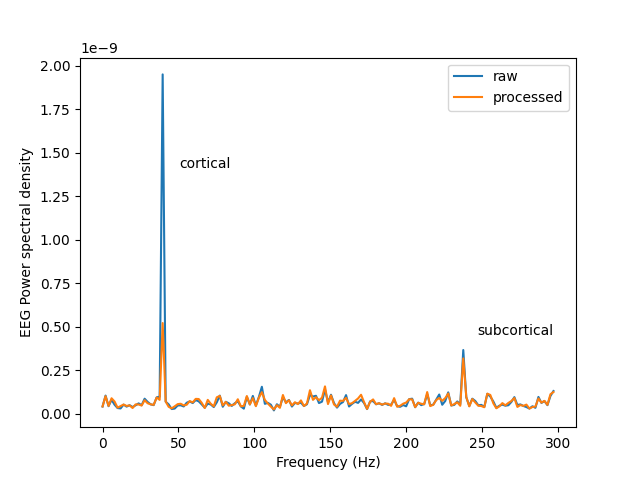# Cortical Signal Suppression (CSS) for removal of cortical signals¶

This script shows an example of how to use CSS 1 . CSS suppresses the cortical contribution to the signal subspace in EEG data using MEG data, facilitating detection of subcortical signals. We will illustrate how it works by simulating one cortical and one subcortical oscillation at different frequencies; 40 Hz and 239 Hz for cortical and subcortical activity, respectively, then process it with CSS and look at the power spectral density of the raw and processed data.

```# Author: John G Samuelsson <johnsam@mit.edu>

import numpy as np
import matplotlib.pyplot as plt

import mne
from mne.datasets import sample
from mne.simulation import simulate_sparse_stc, simulate_evoked
```

```data_path = sample.data_path()
subjects_dir = data_path + '/subjects'
fwd_fname = data_path + '/MEG/sample/sample_audvis-meg-eeg-oct-6-fwd.fif'
ave_fname = data_path + '/MEG/sample/sample_audvis-no-filter-ave.fif'
cov_fname = data_path + '/MEG/sample/sample_audvis-cov.fif'
trans_fname = data_path + '/MEG/sample/sample_audvis_raw-trans.fif'
bem_fname = subjects_dir + '/sample' + '/bem' + '/sample-5120-bem-sol.fif'

fwd = mne.convert_forward_solution(fwd, force_fixed=True, surf_ori=True)
fwd = mne.pick_types_forward(fwd, meg=True, eeg=True, exclude=raw.info['bads'])
```

Out:

```Opening raw data file /home/circleci/mne_data/MNE-sample-data/MEG/sample/sample_audvis_raw.fif...
Read a total of 3 projection items:
PCA-v1 (1 x 102)  idle
PCA-v2 (1 x 102)  idle
PCA-v3 (1 x 102)  idle
Range : 25800 ... 192599 =     42.956 ...   320.670 secs
Computing patch statistics...
[done]
Computing patch statistics...
[done]
Desired named matrix (kind = 3523) not available
Read MEG forward solution (7498 sources, 306 channels, free orientations)
Desired named matrix (kind = 3523) not available
Read EEG forward solution (7498 sources, 60 channels, free orientations)
MEG and EEG forward solutions combined
Source spaces transformed to the forward solution coordinate frame
Average patch normals will be employed in the rotation to the local surface coordinates....
Converting to surface-based source orientations...
[done]
364 out of 366 channels remain after picking
366 x 366 full covariance (kind = 1) found.
Read a total of 4 projection items:
PCA-v1 (1 x 102) active
PCA-v2 (1 x 102) active
PCA-v3 (1 x 102) active
Average EEG reference (1 x 60) active
```

Find patches (labels) to activate

```all_labels = mne.read_labels_from_annot(subject='sample',
subjects_dir=subjects_dir)
labels = []
for select_label in ['parahippocampal-lh', 'postcentral-rh']:
labels.append([lab for lab in all_labels if lab.name in select_label])
hiplab, postcenlab = labels
```

Out:

```Reading labels from parcellation...
```

Simulate one cortical dipole (40 Hz) and one subcortical (239 Hz)

```def cortical_waveform(times):
"""Create a cortical waveform."""
return 10e-9 * np.cos(times * 2 * np.pi * 40)

def subcortical_waveform(times):
"""Create a subcortical waveform."""
return 10e-9 * np.cos(times * 2 * np.pi * 239)

times = np.linspace(0, 0.5, int(0.5 * raw.info['sfreq']))
stc = simulate_sparse_stc(fwd['src'], n_dipoles=2, times=times,
location='center', subjects_dir=subjects_dir,
labels=[postcenlab, hiplab],
data_fun=cortical_waveform)
stc.data[np.where(np.isin(stc.vertices, hiplab.vertices)), :] = \
subcortical_waveform(times)
evoked = simulate_evoked(fwd, stc, raw.info, cov, nave=15)
```

Out:

```Projecting source estimate to sensor space...
[done]
4 projection items deactivated
Created an SSP operator (subspace dimension = 4)
4 projection items activated
SSP projectors applied...
```

Process with CSS and plot PSD of EEG data before and after processing

```evoked_subcortical = mne.preprocessing.cortical_signal_suppression(evoked,
n_proj=6)
chs = mne.pick_types(evoked.info, meg=False, eeg=True)

psd = np.mean(np.abs(np.fft.rfft(evoked.data))**2, axis=0)
psd_proc = np.mean(np.abs(np.fft.rfft(evoked_subcortical.data))**2, axis=0)
freq = np.arange(0, stop=int(evoked.info['sfreq'] / 2),
step=evoked.info['sfreq'] / (2 * len(psd)))

fig, ax = plt.subplots()
ax.plot(freq, psd, label='raw')
ax.plot(freq, psd_proc, label='processed')
ax.text(.2, .7, 'cortical', transform=ax.transAxes)
ax.text(.8, .25, 'subcortical', transform=ax.transAxes)
ax.set(ylabel='EEG Power spectral density', xlabel='Frequency (Hz)')
ax.legend()

# References
# ^^^^^^^^^^
#
# .. footbibliography::
```Total running time of the script: ( 0 minutes 5.342 seconds)

Estimated memory usage: 100 MB

Gallery generated by Sphinx-Gallery